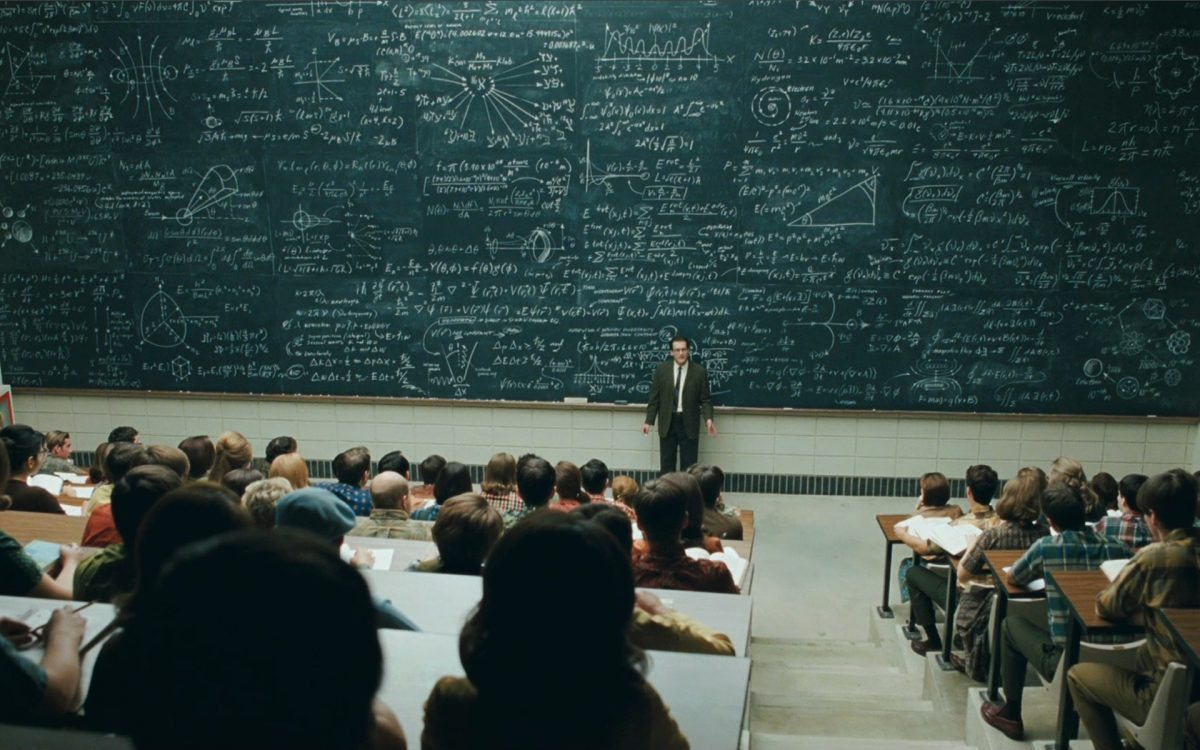# An Elegant Weapon

In Mathematics by Brian Koberlein6 Comments

Mathematics is the language of science. From arithmetic to group theory, mathematics builds the very foundation of scientific models. We might be inspired by an idea or analogy, but the precision of science requires a mathematical structure. Perhaps the most fundamental thing we’ve learned about the cosmos is that it has a deep connection to mathematics.

This connection has raised the question about just why mathematics is so effective. Perhaps it’s simply due to the fact that we follow mathematical models where they are useful, and discard them where they are not, making applied mathematics self-selecting. Perhaps it’s because as evolved primates within this physical universe the mathematics we think is “pure” is simply a reflection of how our universe works. Regardless of the cause, mathematics seems unreasonably effective as physicist Eugene Wigner once argued. It’s so powerfully useful that some folks such as Max Tegmark have proposed that the structure of the Universe could simply be the structure of mathematics itself. Gallons of ink have been spilled on all sides.

But the very power of mathematical models within science also raises a wall separating those with the mathematical training to understand these models from those without. This is particularly seen in the popularization of science where (with some exceptions) equations never appear, and the focus is on broad analogies rather than the underlying maths. This reinforces the misconception that established science can be overturned simply be a new idea, and that the mathematics is merely a minor detail. The reality is that an idea can be a spark, but fire of knowledge is only captured with specific mathematics, and it’s in the mathematics where much of the beauty an elegance of a scientific model lies. The mathematics of science can be deep and subtle, and its nature is not often discussed.

So for the next several posts I’ll try to present some of the mathematical beauty behind several scientific theories:

1. Geometry – From Aristotle’s conception of earthly lines and heavenly circles, to Kepler’s elliptical forms, geometry has played a central role in astronomy. As we followed geometry into more abstract concepts, it opened the doors to the beginning of time.
2. Vectors and Fields – Forces have both a quantity (magnitude) and direction. In mathematics we call them vectors. From that simple mathematical concept arose the first unified field theory.
3. Complex Numbers – It was long thought that any number multiplied by itself is a positive number. But what if a number multiplied by itself was negative? This idea was so odd it came to be known as imaginary. It turns out that imaginary numbers open the door to very real physics.
4. Group Theory – We often think of mathematics as numbers, or at least equations. But it can also be about relationships and connections. Often how different parts of a model connect is the key to understanding the model on a deeper level.
5. Formalism – Is mathematics simply a set of connected rules, or is it something more? Does mathematics limit what we can know about the physical universe?

We’ll start with geometry, and how a simple curve tracing the motion of a planet led us to an exploration of space and time itself. It all begins tomorrow.

Paper: Wigner, E. P. The unreasonable effectiveness of mathematics in the natural sciences. Communications on Pure and Applied Mathematics. 13: 1–14 (1960). DOI:10.1002/cpa.3160130102

Paper: Max Tegmark. Is “the theory of everything” merely the ultimate ensemble theory? arXiv:gr-qc/9704009 (1998).

1.Wigner’s essay is a time tested classic.

2.Mathematics is the dark chocolate of science. It is marrow for the brain. ?

3.Awesome…watched NOVA The Great Math Mystery the other day…will be cool getting a bit deeper…

4.Sorry, but my Aspergers is kicking in again. How did that professor get all of those elegant equations and drawing so high uo on the chalkboard? Did he use a ladder? Does the chalkboard move up and down? Is it merely wallpaper to intimidate and weed out inferior students? This is why I suck at math; too distracted by too many distractions I guess. I do thoroughly enjoy your posts though, but sadly I will never have a true mathematical understanding of the topics you cover 🙁

5.I think that blackboard scene is from a movie. The blackboard does not look movable. Movable blackboards are usually segmented, otherwise you’d never be able to get the top down where you can write on it.

I’d attribute the picture to someone wanting to impress theatre-goers.

6.He used a ladder stand that rolls ,almost like a scaffle …..